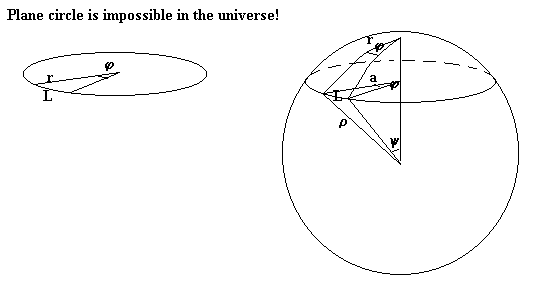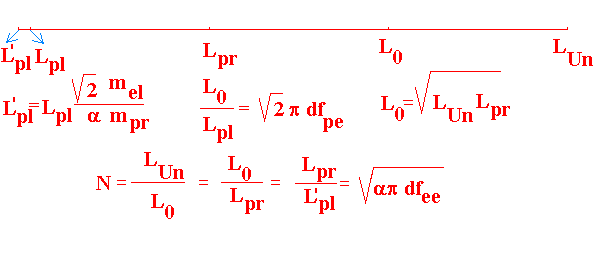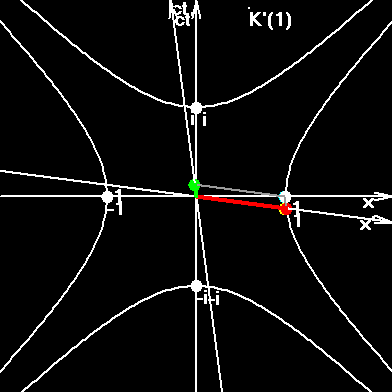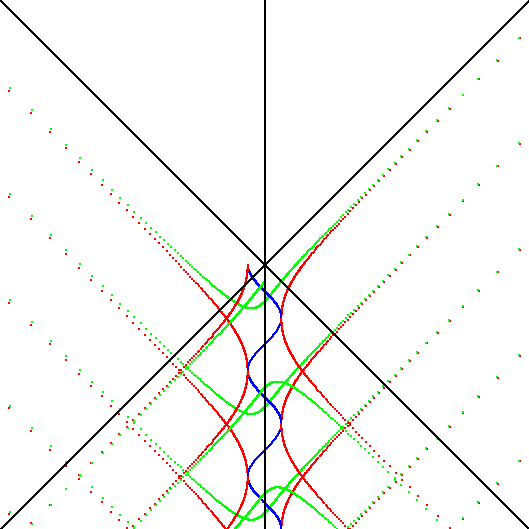# NORMALIZED UNITS

It is supposed in this work that there exist three types of 4-dimensional rotation in the Universe.

• Rotation of Universe as a whole. Let's name it grand or universal rotation. Don't mix this grand 4-d rotation with usual three dimensional rotation. One full grand rotation takes time: T=1/H. We'll denote it ROT.
• Most hard to imagine, but nevertheless existing 4-d rotation on our scale of space-time. This rotation reduces non isotropic space-time lattice to isotropic. Observable time becomes here as a successive set of "flashes" - materialization of a matter after every full space-time "flash", or middle rotation. Space and time intervals, and frequencies are measured here in the same units, - "flashes", or "steps", or middle rotations. Let's denote this new unit by "!".
• Rotation of space-time in the microworld. This rotation is described by Planck constant and by the most massive stabile particle - proton. One full rotation here corresponds to the Compton wave length of proton. Let's denote this unit osc (oscillation).

1 ROT = N !, 1! = N osc, 1 ROT = N2 osc, !2 = sqr(ROT*osc)

In order to simplify relations between values we'll introduce mass of proton pr as unit of mass, and charge of an electron e as unit of charge.

We don't know the exact geometry of the real Universe. So, we can speak about it's space-time coordinates only with some approximation. Today, it is clear, that in the present geometrical model of Universe, the coordinates of 4-d lattice are almost closed, i.e., after finishing one full rotation about the Universe, the coordinate axis-circumference is not closed, but passes close to initial point, - about the Compton wavelength of proton. By taking this into account, we can write that the length of one quasi spire is defined through velocity of light and Hubble constant as:

X = c/H (1)

The length of time quasi spire is:

T = 1/H (2)

H - Hubble constant. Observations give for it H = 70 +/- 15 km/s/Mpc. In this work the following result was received: H= 73.29 km/s/Mpc, and after corrections of units H = 2.375E-18 ROT/s. The length of space and time spires of Universe are: X= 1.262E+26 m/ROT, T = 4.210E+17 s/ROT or T = 13.34 mlrd.years/ROT.

The expressions (1), (2) are valid in 4-d lattice from the point of view of macroscopic observer, i.e., relatively us and our units. In this units the lattice looks non symmetrically, - three quasi spires numerically are big, the fourth is shorter by "c" times. By making the turn inside the lattice, we at the same time make equal all quasi spires. (To say the truth, the macroscopic life is impossible under such angles, because there will be no difference between proton, electron, photon, neutrino, because there will no be time with all thermodynamics, and no accumulation of information.) Nevertheless the microscopic life is possible there and we can assume that the length of all quasi spires are equal to each other and numerically are equal to some universal quantum number.

" ' "- denotes new normalized values of our Universe. At first N was received from expression N = sqr(c2mpr/(Hh), but the precision of Hubble constant was not sufficient, and latter the N was received more precisely through another expressions. Now we have N = 3.091E+20 !/ROT.

Here is the system of equations with which we can receive exact values of quantities.

1. The connection of quantum number N, with the length of space X and time T quasi spires of Universe, with velocity of light c, Hubble constant H, boundary frequency n0, boundary wave length l0 and boundary time interval t0 in SI: N=Tn0, N=X/l0, X = c/H, T = 1/H, t0 = 1/n0.
2. The same connection in Normalizes Units (NU): N=X'=T'=1/H'.
3. Connection with mimimum H and maximum mprc2/h frequencies in the Universe: N=sqr(mprc2/hH),
4. Connection with ratio of forces: N = sqr(ap(fel/fgr)e-e), where (fel/fgr)e-e is the ratio of electric to gravity interaction between two electrons; a - fine structure constant.

Note: In the summer 1999, the precision of all recommended values was changed by CODATA. Only one of the main constants had become less precise, -- gravity constant. It was changed from 6.67259(85)E-11 to 6.673(10)E-11, or in form, used in this work: from 6.67259E-11 to 6.673E-11 in SI units. In this work a very interesting numerical coincidence was found for normalized gravity constant G' = 1/Exp(a+1/a) and, as a result, we received very precise G = 6.671479888E-11. It does not influence on the main results of normalization of units, but great precision of G helps us to see more clearly the beauty of normalized units. So if hypothesis G' = 1/Exp(a+1/a) will be erroneous, then precision of some bottom results will be less. Like: from 6.671479888E-11 to 6.673E-11. This will not change the main results of normalization.

Let's introduce the main coefficients of re-count of space and time quantities from SI to NU, and coefficients of re-count of mass and charge. The unit of mass in NU is the mass of proton. The unit of charge in NU is the elementary charge.

 Connected quantity Coefficient of re-count Value of SI unit in NU Time t0 = 1.362402672E-03 s/! 1 s = 733.9973861 ! Length l0 = 408438.0458 m/! 1 m = 2.44863066E-06 ! Mass km = 1.67262158E-27 kg/pr 1 kg = 5.978638624E+26 pr Charge q = 1.602176462E-19 C/e 1 C = 6.2415097445E+18 e

Let's introduce coefficients for re-count of frequencies:

 Frequency of oscillations in zt n0 = 733.9973861 !*Hz 1Hz (oscill/s) = 1.362402672E-03 1/! (oscill./flash) Frequency of rotations in xy n0 = 733.9973861 !/s 1/s (rot/s) = 1.362402672E-03 1/! (rot/flash)

Velocity of light is dimensionless in NU and equals to one: c' = c/(l0n0) = 299792458m/s/(408438.0458 m/!*733.9973861 !/s) = 1

Hubble constant:

H'=1/T'=1/N = 2.032783924E-21(universal rotation / flash) = 3.235276097E-21 ROT/! (4)

In SI units Hubble constant will be interpreted as angular velocity of light: H = 2.374684198E-18 (universal rotation / second) = 2.374684198E-18 (ROT/s), H*(2p) = 1.492058087E-17 (universal radian / second) = 1.492058087E-17 (RAD/s).

Normalized unit of force will be proton-force or pr-force or pr/!: 1pr-force =1pr*1!/1!/1!= 1pr/!. 1N = 1kg*1m/1s2/ 1.67262158E-27 kg/pr / 408438.0458 m/! * (1.362402672E-03 s/!)2 = 2.716984040E+15 pr/!, (or 1N =2.716984040E+15 pr-force.

kF=3.680551617E-16 N/pr-force (or in N*!/pr) (5)

Normalized unit of energy is also proton: 1 J = (1kg*1m2/1s2)/km /l02*t02 = 6652132600 pr or 1 pr = 1.503277310E-10J.

kE = 1.503277310E-10 J/pr (6)

The Planck constant will be:

h' = {h}(J*s/osc) * {n0}(!/s) / {kE}(J/pr)  = 3.235276097E-21 pr*!/osc (7)

Compton wavelength of proton:

lpr = h / (mprc) = 1.321409846E-15 m/osc (8)

lpr' = {lpr}(m/osc) / l0(m/!) = 3.235276097E-21 !/osc (8.a)

Gravity constant G in normalized form:

G' = {G}(m3ROT/(s2kg))*({t0}(s/!))2*{km}(kg/pr)/({l0}(m/!))3 = 3.039850897E-60 !*ROT/pr (9)

(The course of intrusion of ROT in the gravity constant will be explain later)

As a result we have long and not finished yet chain of numerical exact equalities:

{X'}={Y'}={Z'}={T'}=1/{h'}=1/{H'}=1/v0'=1/{l'pr}=1/{f'}={N}=3.090926308E+20 (10)

where f' is quantum of force, and v0' is quantum of velocity of particle's link (quark).

### Quantum of force and graviton energy.

Energy of fluctuations must have symmetry form from both sides of the boundary frequency. Such for fluctuation, lying in the electromagnetic region, or simply, for photon, we have:

E = hn = hc/l (11)

Correspondingly, the energy of fluctuation, lying in the space-gravity region, or simply, for graviton, must be defined through:

E = fl = fc/n (12)

where f is quantum of force, defined from the condition of correctness of both formulas at the border: Quantum of force must play the same role in gravity region, as quantum of action plays in electromagnetic region.

E0=hn0=hc/l0=(hc/l02)l0 (13)

f = hc/l02 (14)

After substituting of dates and using equations osc/!=!/ROT, !2=osc*ROT, we'll receive:

f = {h}(J*s/osc)*{c}(m/s)/({l0}(m/!))2 = 1.190760067E-36 N*ROT

After multiplying the last by 2p (RAD/ROT) we'll receive analogue to hbar:

We can take ratio of action quantum to force quantum:

h/f=556.4570849 (J*s/osc) / (N*ROT), or m*s/!2 (15)

Expression (15) is equal to: l0/n0 = 556.4570849 (m/!)/(!/s), or m*s/!2 (16)

Expression (15.b) quite strangely connects time, linear, angular quantities in SI and NU: 1radNU = sqr(14.09522262 m*s) = 3.7543604810(m*s)1/2

In normalized units the quantum of force is equal to 1/N pr*ROT/!.

### Normalization of electric units.

Electric constant, or permittivity, e0 can be received from the expression for elementary charge: e=sqr(2ae0hc), or: e'=sqr(2ae0'h')

e0'=e'2/(2ah')=N/(2a) e2osc/(pr*!) = 2.117840884E+22 e2osc/(pr*!) (17)

The tests of dimensions in different expressions had shown, that dimensions of constants of interactions are dependent of units ROT or osc. For dimensions of gravity constant we have [G] = 1kg*s2*ROT/m3. We can conclude that G, H are dependent of the angle of rotation in lattice of Universe. But it is supposed in this work that the angle does not dependent of time. Our situation in universal space-time lattice is analogues to the height of a running squirrel in rotating while.

Precision of values N, H, l0, n0, t are defined by the precision of gravity constant G. But it's precision is the smallest among main constants. If we take combinations of N, H, l0, n0, t with G, then precision will be much bigger, for example:

G=6.673E-11 ROT*m3/(kg*s2), H=2.375E-18 ROT/s, G/H=2.809417729E+7 m3/kg/s (18)

G/H can be interpreted as a specific "swallowing" of material space by ponderable matter.

Permeability of free space in normalized units can be received from: c=1/sqr(e0m0), 1=1/sqr(e0'm0'), m0'=1/e0'.

m0'= 2a/N = 4.721790044E-23 pr*!/osc/e2 (19)

Impedance for vacuum in SI is Z0=sqr(m0/e0)=376.730313461771 Ohm, and in norm. units it is:

Z'=sqr(m0'/e0')=m0'=2a/N= 4.721790044E-23 pr*!/osc/e2 (20)

### Summary table

 Value in SI Re-count Value in NU Quantum number N N=T*n0, N=X/l0, N=sqr(mprc2/hH), N=sqr(ap(fel/fgr)e-e), N=3.090926308E+20 osc/!, !/ROT, sqr(osc/ROT) N'=N 3.090926308E+20 osc/!, !/ROT, sqr(osc/ROT) Velocity of light c = 299792458 m/s c'=ct0/l0 1 Hubble constant H = 2.374684198E-18 ROT/s 73.275107 km/s/Mpc H'=Ht0 = 1/N 3.235276097E-21 ROT/! Compton wave length of proton lpr = h / (mprc) =1.321409847E-15 m/osc lpr' = lpr/l0 = 1/N 3.235276097E-21 !/osc Compton frequency of proton npr = 2.268731830E+23 osc/s npr'=nprt0 =N 3.090926308E+20 osc/! Planck constant h= 6.626068756E-34 J*s/osc h' = h/km/l02t0 = 1/N 3.235276097E-21 pr*!/osc Electron mass mel= 9.109381876E-31 kg mel'=mel/km 1/1836.1526675 pr Gravity constant G = 6.671479888E-11 ROT*m3/(kg*s2) G' = Gt02km/l03 ; G' =N/p2*df2/2 (independent hypothesis: G'= 1/Exp(a+1/a), or 2G'= 1/sh(a+1/a), or 2G'= i/sin(i(a+1/a))) 3.039850897E-60 !ROT/pr Quantum of force f = hc/l02 = 1.190760067E-36 N*ROT or kg*m*ROT/s2 f' = ft02/(kml0) ={1/N} 3.235276097E-21 pr*ROT/! Electric constant e0=8.85418782E-12 F/m, C2osc/(m2N) e0' =e'2/(2ah') = {N/(2a)} 2.117840884E+22 e2osc/!/pr Magnetic constant m0=1/c2e0= 4p*E-7 Hn/(osc*m), s2N/(osc*C)2 m0' = 1/e0' = {2a/N} 4.721790044E-23 pr*!/osc/e2 Impedans of free space Z0=sqr(m0/e0) = 376.730313461771 Ohm/osc, m*s*N/(osc*C2) Z0' = sqr(m0'/e0') {2a/N} 4.721790044E-23 pr*!/osc/e2 Ratio of coefficients of interactions grav/el d = G4pe0 = 7.423022472E-21 C2ROT*osc/kg2, C2!2/kg2 d' = d*km2/ke2 8.090129511E-37 e2!2/pr2 Grav/el forces ratio between two protons dpp=d*mpr2/q2 = 8.090129511E-37 !2 dpp' = dpp 8.090129511E-37 !2 Grav/el forces ratio between proton and electron dpe=d*mpr*mel/q2 =4.406022252E-40 !2 dpe' = dpe 4.406022252E-40 !2 Grav/el forces ratio between two electrons dee=d*mel2/q2 = 2.399594723E-43 !2 dee' = dee = ap/N2 2.399594723E-43 !2

In SI we have seven base quantities: length (meter, m); mass (kilogram, kg); time (second, s); electric current (ampere, A); thermodynamic temperature (kelvin K); amount of substance (mole, mol); luminous intensity (candela, cd). Other quantities can be represented as additional quantities and derived quantities. Examples of additional quantities are: plane angle (radian, rad); solid angle (steradian, sr). Examples of derived quantities are: mass density (kilogram per cubic meter, kg/m3); energy (joule, J=N*m=m2*kg*s-2); power (watt, W=J/s=m2*kg*s-3); charge (coulomb, C=A*s); and so on. Plane and solid angle are dimensionless and this is the course of some confusion in the formulas with "rad" and "sr".

It is clear, that apparent disorder with units is bound to closed geometry. Such similar "disorder" exists in elementary math. For example, the length of arc, L, and the length of it's radius, r, are measured in "meters". The angle, j is measured in "radians". We have an equation:

L (m)=r (m) * j (rad) (21)

As a result we have 1m = 1m*1rad. Because of this correct/noncorrect equation we have two different Planck constant, related by the coefficient 2p but both measured in J*s.

We can change the approximate equation (21) by more precise with the help of bottom picture and constituting the fact, that in the real world there are no straight lines, there are no plane circles, there is no ideal Euclidean space.Any circumference or circle we must always draw on the sphere of much more radius. So, from L=aj, a= rsiny, r=ry we can receive more precise formulae for the length of arc:

The equation (22) now is expressed without contradictions in units: m = m*rad/rad, siny = m/m. But to be correct to the end we must rewrite the expression, containing the radius of curvature r, in correct form: r=rysind/d. The result will be the same.

From the other hand the formulae L=rJ, where J = jsiny/y, can be regarded as correct, but in this case the quantity J must be named not angle, but the ratio of lengths. Correspondingly in formulae: r=rY, where Y=ysind/d, the quantity, denoted by big Y will be the ratio of arc length to radius length, but the quantity, denoted by small y is the angle of this arc, measured in radians.

### Example transition from photon to graviton.

In the border n0 = 734 !/s the properties of oscillations are changed relatively us, macroscopioc observers, who live at the scale story between microworld and megaworld. At this frequency we must change space measurements on time measurement and visa verse. It is possible to do this in normalized units. For example, let's suppose, that "mirror reflection" of maximum of cosmic microwave background radiation (CMBR) can give us the some maximum gravity spectrum. Then, transferring from usual units to normalized, and returning from normalized units to usual we'll have such chain of lines, where osc - electromagnetic oscillations, ROT - gravity oscillations:

grav. max --- border --- CMBR.max

---- x (Hz or ROT/s)-------- 734 !/s---- 2.82E+11 (Hz or osc/s)

---1.91E-6 ROT/s-----------734 !/s--------2.82E+11 osc/s---

Last numerical value is the extremum gravity wavelength in the gravity spectrum. For the period of that wave we have:

T = 1/(1.687E-6 ROT/s)= 6.06 days/ROT

It is well known that Sun has whole spectrum of vibrations or periodicities, comparable to now received result. It seems that even origin of seven day humane week is not accidental. The weather on Earth has many periods like now received. Besides, the most widespread universe's "lighthouses" have the period close to this.

### Planck values

In the bottom picture You can see the connections of Planck length with now received results in logarithmic scale. (Planck's time and mass one can receive through: tpl=lpl/c; npl=1/tpl; mpl=hnpl/c2)df - el/grav forces ratio for:
pe - proton-electron, ee - electron-electron.

To index of Space Genetics.

Ivan Gorelik,

My VB-program SR2007.exe proves that the electron is not a point, but a string, embracing the whole Universe in a period, equal to electron's classic period.Particles sew and stitch the space-time, constantly recharging electric and colour field, which are constituent subspaces of our whole macroscopic space-time.Interested? Then go to the index of Space Genetics